International
Tables for
Crystallography
Volume F
Crystallography of biological macromolecules
Edited by M. G. Rossmann and E. Arnold

International Tables for Crystallography (2006). Vol. F, ch. 11.1, p. 209   | 1 | 2 |

## Section 11.1.2. The crystal orientation matrix

M. G. Rossmanna*

aDepartment of Biological Sciences, Purdue University, West Lafayette, IN 47907-1392, USA
Correspondence e-mail: mgr@indiana.bio.purdue.edu

### 11.1.2. The crystal orientation matrix

| top | pdf |

The position x (x, y, z) of a reciprocal-lattice point can be given as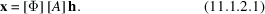The matrix [Φ] is a rotation matrix around the camera's spindle axis for a rotation of ϕ. The vector h represents the Miller indices (h, k, l) and [A] defines the reciprocal unit-cell dimensions and the orientation of the crystal lattice with respect to the camera axes when ϕ = 0. Thus,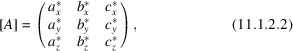where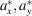and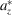are the components of the crystalaxis with respect to the orthogonal camera axes. When an oscillation image is recorded, the position of a reciprocal-lattice point is moved fromto, corresponding to a rotation of the crystal fromto. The recorded position of the reflection on the detector corresponds to the point x when it is on the Ewald sphere somewhere betweenand. The actual value of ϕ at which this crossing occurs cannot be retrieved from the oscillation image. We shall therefore assume here, as is the case in all other procedures, that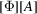defines the crystal orientation in the centre of the oscillation range. Defining the camera axes as in Rossmann (1979), it is easy to show that a reflection recorded at the position (X, Y) on a flat detector normal to the X-ray beam, at a distance D from the crystal, corresponds to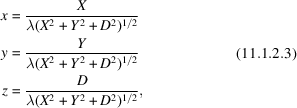where λ is the X-ray wavelength.

If an approximate [A] matrix is available, the Miller indices of an observed peak at (X, Y) can be roughly determined using (11.1.2.3) and (11.1.2.1), wherewith the error being dependent upon the width of the oscillation range, the error in the detector parameters and errors in determining the coordinates of the centres of the recorded reflections.

### References

Rossmann, M. G. (1979). Processing oscillation diffraction data for very large unit cells with an automatic convolution technique and profile fitting. J. Appl. Cryst. 12, 225–238.